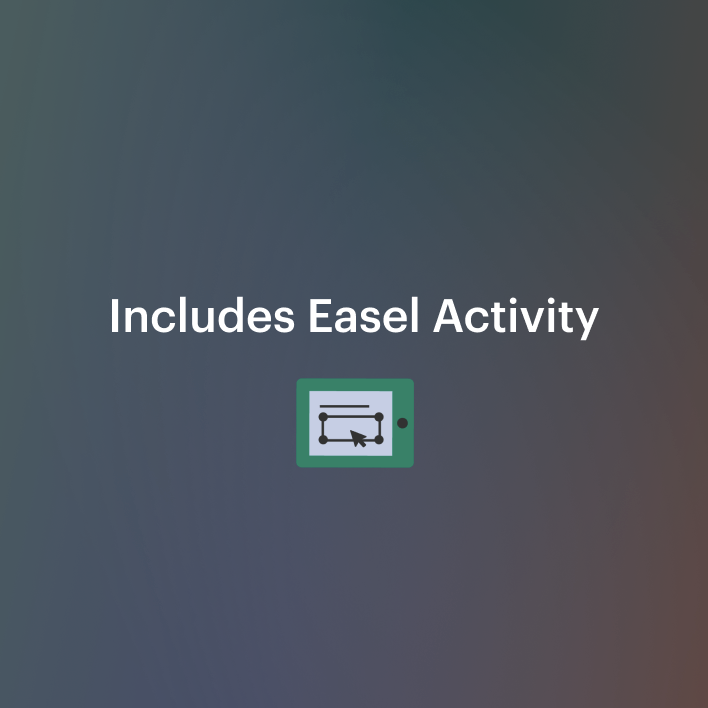# 3rd Grade Multiplication Word Problems Worksheets Minecraft | Google Classroom3rd - 4th, Homeschool
Subjects
Standards
Resource Type
Formats Included
• Zip
•Google Apps™
•Activity
Pages
43 pagesThe Teacher-Author indicated this resource includes assets from Google Workspace (e.g. docs, slides, etc.).
Easel Activity Included
This resource includes a ready-to-use interactive activity students can complete on any device. Easel by TpT is free to use! Learn more.

### Description

These 3rd grade multiplication worksheets are perfect for your Minecraft-loving students! This word problem resource has been updated to include interactive Google™️ Slides!

If your students love Minecraft, but are not interested in multiplication, these multiplication word problems with answers will be a winner! You will be able to tap into the excitement of the game and transfer that feeling to multiplication.

There are 4 versions of this resource to meet the needs of all your learners → in person, distance learning, or hybrid.

• ➤ Interactive Google™️ Slides
• ➤ Printable color PDF
• ➤ Printable blackline PDF
• ➤ Interactive Easel activity on TpT

Each multiplication word problem is based on a scenario that could happen while playing Minecraft and provides practice with bar models, number bonds, & number lines to represent multiplication through creating, constructing, and deconstructing.

TO MAKE YOUR LIFE EASIER & TO SAVE YOU TIME YOU'LL FIND...

• ⭐ Answer keys for EVERY problem
• ⭐ Detailed explanations for each problem
• A step by step "Directions" video that walks students through how to use the interactive elements in the Google™️ Classroom including...dragging and dropping, typing in text boxes, & adding arrows to a number line

Perfect for:

• ➤ Distance Learning
• ➤ Google™️ Classroom
• ➤ Small Groups
• ➤ Math Stations or Centers
• ➤ Independent Practice
• ➤ Morning Work
• ➤ Spiral Review

Other Multiplication Products:

More Helpful Math Resources:

Do you like FREEBIES?

Visit me on my blog, Happy Teacher Mama, and sign up to gain FREE LIFETIME ACCESS to my Printable Library! Each printable has a blog post link showing you how I use each one, and the library is consistently updated to give you more amazing FREE content!

Thank you for stopping by my store!

❀ Jennifer

Follow Me on Pinterest

Follow Me on Instagram

Total Pages
43 pages
N/A
Teaching Duration
2 Weeks
Report this resource to TpT
Reported resources will be reviewed by our team. Report this resource to let us know if this resource violates TpT’s content guidelines.

### Standards

to see state-specific standards (only available in the US).
Interpret products of whole numbers, e.g., interpret 5 × 7 as the total number of objects in 5 groups of 7 objects each. For example, describe a context in which a total number of objects can be expressed as 5 × 7.
Interpret whole-number quotients of whole numbers, e.g., interpret 56 ÷ 8 as the number of objects in each share when 56 objects are partitioned equally into 8 shares, or as a number of shares when 56 objects are partitioned into equal shares of 8 objects each. For example, describe a context in which a number of shares or a number of groups can be expressed as 56 ÷ 8.
Use multiplication and division within 100 to solve word problems in situations involving equal groups, arrays, and measurement quantities, e.g., by using drawings and equations with a symbol for the unknown number to represent the problem.
Determine the unknown whole number in a multiplication or division equation relating three whole numbers. For example, determine the unknown number that makes the equation true in each of the equations 8 × ? = 48, 5 = __ ÷ 3, 6 × 6 = ?.
Apply properties of operations as strategies to multiply and divide. Examples: If 6 × 4 = 24 is known, then 4 × 6 = 24 is also known. (Commutative property of multiplication.) 3 × 5 × 2 can be found by 3 × 5 = 15, then 15 × 2 = 30, or by 5 × 2 = 10, then 3 × 10 = 30. (Associative property of multiplication.) Knowing that 8 × 5 = 40 and 8 × 2 = 16, one can find 8 × 7 as 8 × (5 + 2) = (8 × 5) + (8 × 2) = 40 + 16 = 56. (Distributive property.)

### Questions & Answers

Teachers Pay Teachers is an online marketplace where teachers buy and sell original educational materials.